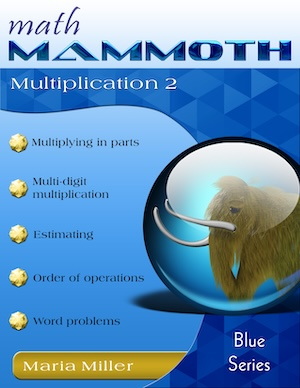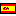^
You are here: HomeBlue Series → Multiplication 2

# Math Mammoth Multiplication 288 pages

Sample pages (PDF)

## Prices & ordering

Printed copy \$9.75Este libro en español

Math Mammoth Multiplication 2 is a worktext focusing on multi-digit multiplication and related topics. It best suits fourth grade mathematics.

The first lessons briefly review the concept of multiplication and the multiplication tables. Next, students encounter equations in disguise — presented with shapes on both sides of a pan balance — in the lesson Scales Puzzles. This lesson is intended to be fun and motivational.

Then, the focus shifts to multi-digit multiplication (also called multiplication algorithm or multiplying in columns). We start out by learning to multiply numbers by multiples of ten and hundred (for example, 20 × 4 or 500 × 6). After this is mastered, students learn the very important concept of multiplying in parts, or partial products. This means that, for example, we multiply 4 × 63 in two parts: first we multiply 4 × 60 = 240 and 4 × 3 = 12, and lastly the results are added: 240 + 12 = 252.

This principle underlies all other multiplication algorithms, so it is important to master. We don’t want children to “blindly” memorize the multiplication algorithm without understanding what is going on with it. The partial products algorithm (multiplying in parts) also ties in with an area model, and it is very important that students see the connection between this visual model and the procedure.

The book contains two lessons about multiplying in columns the “easy way”. This “easy way” is a simplified form of the traditional multiplication algorithm, based on partial products. You may skip these two lessons at your discretion. The method taught in those lessons is most useful for students who may have trouble with the traditional form of the algorithm. This method is also helpful in cementing the student’s understanding of the partial products method.

The traditional, or standard, form of multiplication algorithm is taught next, and is hopefully fairly easy, with the partial products as a foundation.

Students also study estimation, the order of operations, and multiplying with money. There are numerous word problems in all of the lessons. Students are encouraged to write number sentences for the word problems—essentially learning to show their work and their thinking process.

The lesson So Many of the Same Thing has to do with proportional reasoning. The idea is really simple, and prepares students for learning ratios and proportions in middle school.

The last major topic in the book is multiplying two-digit numbers by two-digit numbers. Again, we first study partial products and tie that in with an area model. The lesson Multiplying in Parts: Another Way is optional. Lastly, the book teaches the standard algorithm for two-digit by two-digit multiplication. Students will practice multiplication with more digits in the book Mammoth Multiplication & Division 3.

The PDF version of this book can be filled in on a computer, phone, or tablet, using the annotation tools found in many PDF apps. See more.

You might also be interested in:
• Math Mammoth Division 2—a worktext about long division, word problems, average, and divisibility (grades 4-5).
• Math Mammoth Multiplication & Division 3—a worktext about multi-digit multiplication and long division with larger numbers, ratios, simple equations, divisibility, primes, and factoring (grades 5-6).
• Make It Real Learning: Arithmetic I—an activity workbook with real-world data, focusing on situations that use addition, subtraction, estimation, division, bar graphs, and place value with large numbers (grades 3-6).

## Better Yet - Bundle Deals!

*BONUS*: Buy any bundle listed above, and get the fully upgraded Soft-Pak software for FREE (includes 4 math and 2 language arts programs, complete with on screen and printable options).

## How and where to order

You can buy Math Mammoth books at:

• Here at MathMammoth.com website — simply use the "Add to cart" buttons you see on the product pages.
• Rainbow Resource carries printed copies for the Light Blue series books, plus several CDs (Light Blue and Blue series).
• Homeschool Buyers Club offers download versions of the Light Blue series, plus the Blue series bundle.
• Homeschool Planet sells the digital versions of the main curriculum and lesson plans to go with them.
• Lulu sells printed copies for most of the Math Mammoth materials (various series).

By purchasing any of the books, permission IS granted for the teacher (or parent) to reproduce this material to be used with his/her students in a teaching situation; not for commercial resale. However, you are not permitted to share the material with another teacher.

In other words, you are permitted to make copies for the students/children you are teaching, but not for other teachers' usage.

Math Mammoth books are PDF files. I recommend you use Adobe Reader to view them, including if you use a Mac. You can try other PDF viewers, but they may at times either omit or mess up some of the images.
WAIT!

Receive my monthly collection of math tips & resources directly in your inbox — and get a FREE Math Mammoth book!You can unsubscribe at any time.

### Math Mammoth Tour

Confused about the different options? Take a virtual email tour around Math Mammoth! You'll receive:

An initial email to download your GIFT of over 400 free worksheets and sample pages from my books. Six other "TOURSTOP" emails that explain the important things and commonly asked questions concerning Math Mammoth curriculum. (Find out the differences between all these different-colored series!)

This way, you'll have time to digest the information over one or two weeks, plus an opportunity to ask me personally about the curriculum.
A monthly collection of math teaching tips & Math Mammoth updates (unsubscribe any time)### "Mini" Math Teaching Course

This is a little "virtual" 2-week course, where you will receive emails on important topics on teaching math, including:

- How to help a student who is behind
- Troubles with word problems
- Teaching multiplication tables
- Why fractions are so difficult
- The value of mistakes
- Should you use timed tests
- And more!

A GIFT of over 400 free worksheets and sample pages from my books right in the very beginning.A monthly collection of math teaching tips & Math Mammoth updates (unsubscribe any time)
Enter your email to receive math teaching tips, resources, Math Mammoth news & sales, humor, and more! I tend to send out these tips about once monthly, near the beginning of the month, but occasionally you may hear from me twice per month (and sometimes less often).• A GIFT of over 400 free worksheets and sample pages from my books.